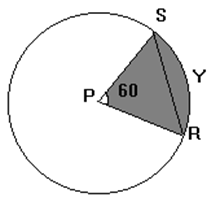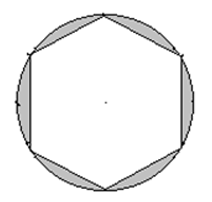# Circles Practice Questions: Level 01

1. What is the radius of a circle if its perimeter is numerically equal to thrice its area?
A. 2
B. 3
C. 2/3
D. 4

2. The area of the sector of a circle, whose radius is 12 metres when the angle at the centre is 60, is
A. 13.2 m2
B. 75.42 m2
C. 13.4 m2
D. None of these

3. Find the radius of the circle if area of sector is 924cm2 and angle at the center is 60.
A. 42
B. 21
C. 22
D. 46

4. The difference between the circumference and area of a circle is 110 metres. Find its circumference.
A. 44m
B. 84m
C. 30m
D. 7m

5. When the radius of a circle is decreased by 100%, the area of the circle:
A. decreases by 50%
B. decreases by 100%
C. increases by 100%
D. decreases by 200%

1. Find circumference of a circle if area of the circle is 154 cm2.
A. 18
B. 22
C. 44
D. 66

2. The difference between the circumference and the diameter of the circle is 60 cm. The area of the circle is:
A. 425 cm2
B. 616
C. 154cm2
D. 308 cm2

3. The circle is 21 cm and m (arc RYS) = 60°. Find the area of the sector.A. 38.5 cm2
B. 231 cm2
C. 25.66 cm2
D. 6.42 cm2

4. A regular hexagon is inscribed in a circle of radius 10 cm. Find the area of the shaded portion. (× ≈ 3.14 ; √3 ≈ 1.73).A. 271 cm2
B. 54.5 cm2
C. 290 cm2
D. 75 cm2

5. The radius of a circle is decreased by 40%. What will be the percentage decrease in its surface area?
A. 64%
B. 36%
C. 20%
D. 10%